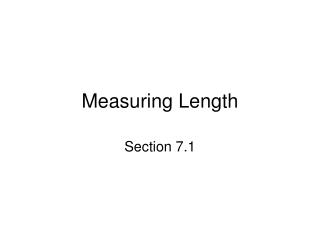DownloadDownload PresentationMeasuring Length

# Measuring Length

Télécharger la présentation## Measuring Length

- - - - - - - - - - - - - - - - - - - - - - - - - - - E N D - - - - - - - - - - - - - - - - - - - - - - - - - - -
##### Presentation Transcript

1. Measuring Length Section 7.1

2. Objectives • Recognize and use appropriate units of length from the English and metric measuring systems • Convert units of length • Unit fractions within the same system • Unit fractions between systems • Moving the decimal point in the metric system • Perform operations on measurements

3. Objective 1:Recognize and use appropriate units of length from the English and metric measuring systems

4. Objective 1 • Unit of measure – name of a quantity that is used as a standard • English system • Metric system

5. Objective 2a:Convert units of length - Unit fractions within the same system

6. Objective 2a • Equivalent measurements – measures of same amount but using different units • Fraction with one unit as numerator and other as denominator is equivalent to 1 (Unit fraction) • Unit fraction converts measurement with one unit to measurement with another unit • Measurement x Unit fraction = Converted measurement • May need to multiply by more than 1 unit fraction to get to final units

7. Objective 2a • To convert units of length • Multiply by unit fraction that has desired units in numerator and original units in denominator • Simplify

8. Objective 2b:Convert units of length - Unit fractions between systems

9. Objective 2b • Use system to system equivalents to write unit fraction • Same method as converting within same system

10. Objective 2c:Convert units of length - Moving the decimal point in the metric system

11. Objective 2c • Metric system based on powers of 10 • Shortcut for metric-to-metric conversions • Multiply or divide by powers of 10

12. Kilometer (km) = 1000 m Hectometer (hm) = 100 m Dekameter (dam) = 10 m Meter (m) = 1 m Decimeter (dm) = 0.1 meter Centimeter (cm) = 0.01 meter Millimeter (mm) = 0.001 meter Objective 2c

13. Objective 2c • To convert units within the metric system • Move decimal point same number of places as number of unit changes

14. Objective 3:Perform operations on measurements

15. Objective 3 • To multiply or divide a measurement by a number • Multiply or divide numbers and write unit of measure • To add or subtract measurements • If units of measure are unlike, convert to like measures • Add or subtract numbers and write unit of measure